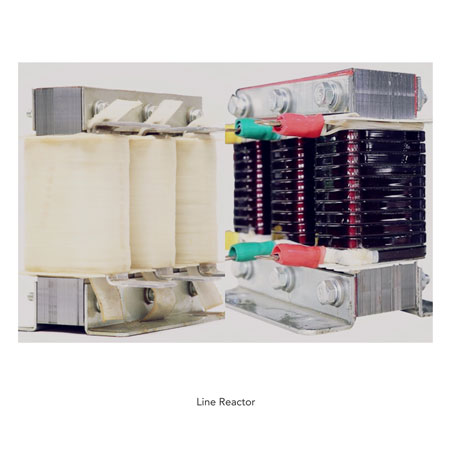Close
(0) Shopping cart
You have no items in your shopping cart.
Shopping Categories
Search

# How to Select a Line Reactor for VFD?

The main function of DC reactor is to reduce the higher harmonic components of the input current, improve the power factors of the input power source, and limit the short-circuit current. Before the DC reactor is connected with the filter capacitor, it hinders the impulse current amplitude entering the capacitor, and reduces the AC impulse of the busbar. When the power of the frequency converter is higher than 22kW, it is suggested to consider the application of DC reactor. The greater the power of the converter is, there should be a higher possibility of using the reactor. Because when there is no DC reactor, the capacitor filtering of the frequency converter will result in the serious distortion of the current wave, thus further causing the serious distortion of the network voltage waveform. In addition, it is very harmful to the service life of the rectifier bridge and filter capacitor of the frequency converter.

Occasions requiring to install the DC reactorWhen the identical power node supplying power to the frequency converter has switching reactive compensation capacitor screen or SCR phase control load, the idle transition caused by capacitor screen switching results in the harmonic wave and power grid waveform gap because of the network voltage mutation and phase control load. It might damage the input rectifying circuit of the frequency converter.

When the unbalanced degree of the power supply three-phase power source of the frequency converter doesn't exceed 3%.

When it is required to improve the power factor of the input terminal of the frequency converter to 0.93 or above.

When the frequency converter is connected with the high-capacity transformer, the current flowing through the input power loop of the frequency converter will probably damage the rectifying circuit.

How to select a line reactor for vfd?
Its calculation and design philosophies are the same as the general reactor. The inductance value of the reactor is appropriate if the voltage drop of the fundamental current flowing through the reactor is not higher than the rated voltage.

Impedance drop: Impedance drop refers to the practical voltage drop on the two terminals of the reactor coil in XHz. Generally, choose impedance voltage drop in 1-4%.

Selection of the inductance value: The rated inductance value of the reactor is an important parameter. If the inductance value is not selected appropriately, it will directly affect the voltage drop change under the rated current, so as to cause fault.

However, the inductance value depends on the sectional area of the reactor iron core, coil winding number and air gap adjustment. The selection of the inductance value of the output reactor is mainly confirmed according to the cable length in the rated frequency scope. And then the sectional areas of the iron core and lead under the corresponding inductance value requirement shall be selected on the basis of the practical rated current of the motor, so as to determine the practical voltage drop.

Inductance value and cable length of the corresponding rated current: cable length rated output current inductance value.

The choice of rated AC current: Rated AC current is to design the long-term working current of the reactor from the perspective of heating. Meanwhile, the sufficient higher harmonic components should be taken into account. In other words, the current practically flowing through the output reactor is the output current of the frequency motor load.